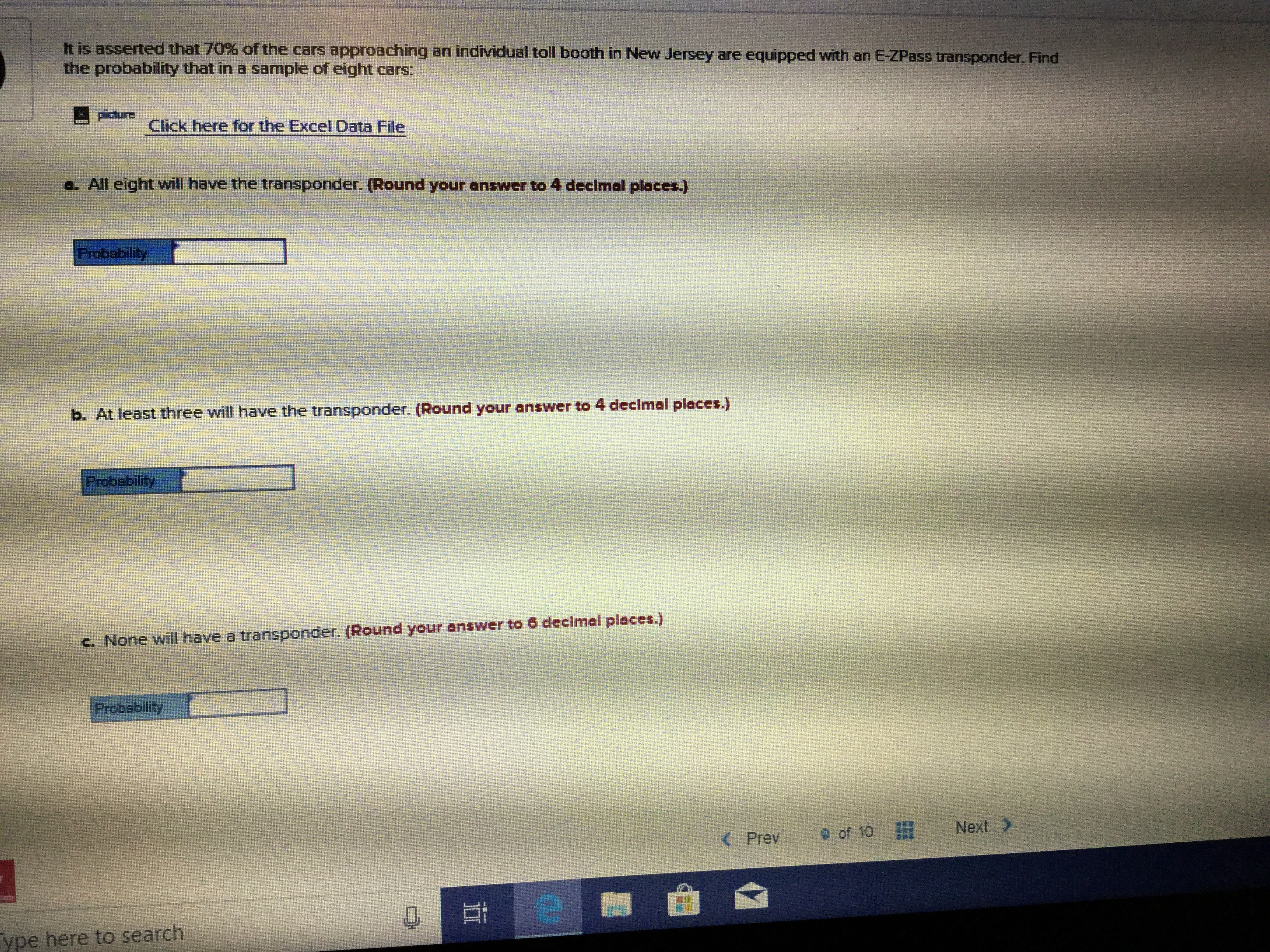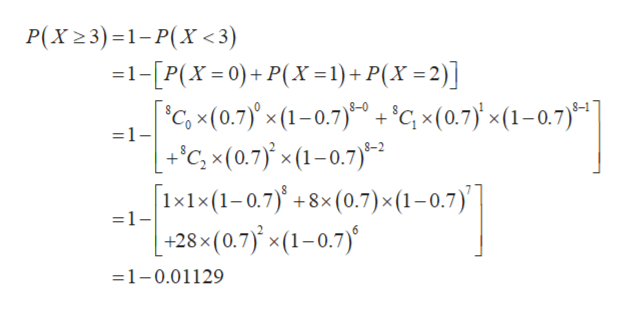# It is asserted that 70% of the cars approaching an individual toll booth in New Jersey are equipped with an E-ZPass transponder. Findthe probability that in a sample of eight cars:PctureவாClick here for the Excel Data Filea All eight will have the transponder. (Round your answer to 4 decimal places.)Frobabilityb. At least three will have the transponder. (Round your answer to 4 decimal places.)Probabilityhave a transponder. (Round your answer to 6 decimal places.)c. None wilProbabilityNext>of 10Prevype here to search

Questionhelp_outlineImage TranscriptioncloseIt is asserted that 70% of the cars approaching an individual toll booth in New Jersey are equipped with an E-ZPass transponder. Find the probability that in a sample of eight cars: Pcture வா Click here for the Excel Data File a All eight will have the transponder. (Round your answer to 4 decimal places.) Frobability b. At least three will have the transponder. (Round your answer to 4 decimal places.) Probability have a transponder. (Round your answer to 6 decimal places.) c. None wil Probability Next> of 10 Prev ype here to search fullscreen
check_circleExpert Solution
Step 1

Given:

Sample size(n)=8

Probabilty(P)=0.70

Explanation:

Let's x is random variable.

The formula of binomial distribution is:

Step 2

The probability that all eight will have the transponder is calculated as follows:

Step 3

Hence, the probability is 0.0576.

b)

To find the probabilty that at least 3 will have the transponder.

Explanation:

The prob...help_outlineImage TranscriptioncloseP(X23)1-P(X<3) 1-[P(X=0)+P(X= 1)+ P(X =2)] 'c, x(0.7)x (1-0.7)'cx(0.7x(1-0.7) +C, x(0.7 x(1-0.7) x1x (1-0.7)+8x (0.7) x (1 - 0.7) -+28x(0.7)'x(1-0.7) =1- =1- 6 =1-0.01129 fullscreen

### Want to see the full answer?

See Solution

#### Want to see this answer and more?

Solutions are written by subject experts who are available 24/7. Questions are typically answered within 1 hour*

See Solution
*Response times may vary by subject and question
Tagged in

### Statistics